# Bihar Board 12th Maths Objective Answers Chapter 13 Probability

Bihar Board 12th Maths Objective Questions and Answers

## Bihar Board 12th Maths Objective Answers Chapter 13 Probability

Question 1.
P has 2 children. He has a son, Jatin. What is the probability that Jatin’s sibling is a brother?
(a) $$\frac{1}{3}$$
(b) $$\frac{1}{4}$$
(c) $$\frac{2}{3}$$
(d) $$\frac{1}{2}$$
(a) $$\frac{1}{3}$$

Question 2.
If A and B are 2 events such that P(A) > 0 and P (b) ≠ 1, then $$P(\bar{A} / \bar{B})=$$
(a) 1 – P(A|B)
(b) $$1-P(A / \bar{B})$$
(c) $$\frac{1-P(A \cup B)}{P(B)}$$
(d) $$\frac{1(\bar{A})}{P(B)}$$
(b) $$1-P(A / \bar{B})$$Question 3.
If two events A and B area such that $$P(\bar{A})$$ =0.3, P(B) = 0.4 and $$P(B | A \cup \bar{B})=$$
(a) $$\frac{1}{2}$$
(b) $$\frac{1}{3}$$
(c) $$\frac{2}{5}$$
(d) $$\frac{1}{4}$$
(d) $$\frac{1}{4}$$

Question 4.
If E and F are events such that 0 < P(F) < 1, then
(a) $$P(E | F)+P(\bar{E} | F)=1$$
(b) $$P(E | F)+P(E | \bar{F})=1$$
(c) $$P(\bar{E} | F)+P(E | \bar{F})=1$$
(d) $$P(E | \bar{F})+P(\bar{E} | \bar{F})=0$$
(a) $$P(E | F)+P(\bar{E} | F)=1$$

Question 5.
P(E ∩ F) is equal to
(a) P(E) . P(F|E)
(b) P(F) . P(E|F)
(c) Both (a) and (b)
(d) None of these
(c) Both (a) and (b)

Question 6.
If three events of a sample space are E, F and G, then P(E ∩ F ∩ G) is equal to
(a) P(E) P(F|E) P(G|(E ∩ F))
(b) P(E) P(F|E) P(G|EF)
(c) Both (a) and (b)
(d) None of these
(c) Both (a) and (b)Question 7.
Two cards are drawn at random one by one without replacement from a pack of 52 playing cards. Find the probability that both the cards are black.
(a) $$\frac{21}{104}$$
(b) $$\frac{25}{102}$$
(c) $$\frac{23}{102}$$
(d) $$\frac{24}{104}$$
(b) $$\frac{25}{102}$$

Question 8.
A bag contains 20 tickets, numbered 1 to 20. A ticket is drawn and then another ticket is drawn without replacement. Find the probability that both tickets will show even numbers.
(a) $$\frac{9}{38}$$
(b) $$\frac{16}{35}$$
(c) $$\frac{7}{38}$$
(d) $$\frac{17}{30}$$
(a) $$\frac{9}{38}$$

Question 9.
Two balls are drawn one after another (without replacement) from a bag containing 2 white, 3 red and 5 blue balls. What is the probability that atleast one ball is red?
(a) $$\frac{7}{15}$$
(b) $$\frac{8}{15}$$
(c) $$\frac{7}{16}$$
(d) $$\frac{5}{16}$$
(b) $$\frac{8}{15}$$

Question 10.
Let A and B be independent events with P(A) = 1/4 and P(A ∪ B) = 2P(B) – P(A). Find P(B)
(a) $$\frac{1}{4}$$
(b) $$\frac{3}{5}$$
(c) $$\frac{2}{3}$$
(d) $$\frac{2}{5}$$
(d) $$\frac{2}{5}$$Question 11.
Two events A and B will be independent, if
(a) A and B are mutually exclusive
(b) P(A’ ∩ B’) = [1 – P(A)] [1 – P(B)]
(c) P(A) = P(B)
(d) P(A) + P(B) = 1
(c) P(A) = P(B)

Question 12.
If A and B are two independent events such that $$P(\bar{A} \cap B)=\frac{2}{15}$$ and $$P(A \cap \bar{B})=\frac{1}{6}$$, then find P(A) and P (B) respectively.
(a) $$\frac{5}{4}, \frac{4}{5}$$
(b) $$\frac{1}{5}, \frac{1}{7}$$
(c) $$\frac{1}{6}, \frac{1}{7}$$
(d) $$\frac{1}{7}, \frac{1}{7}$$
(a) $$\frac{5}{4}, \frac{4}{5}$$

Question 13.
If A and B are two independent events, then the probability of occurrence of at least of A and B is given by
(a) 1 – P(A) P(b)
(b) 1 – P(A) P(B’)
(c) 1 – P(A’) P(B’)
(d) 1 – P(A’) P(b)
(c) 1 – P(A’) P(B’)

Question 14.
If A and B are two indendent events such that $$P(\bar{A})$$ = 0.75, P(A ∪ B) = 0.65 and P(b) = P, then find the value of P.
(a) $$\frac{9}{14}$$
(b) $$\frac{7}{15}$$
(c) $$\frac{5}{14}$$
(d) $$\frac{8}{15}$$
(d) $$\frac{8}{15}$$

Question 15.
If A and Bare events such that P(A) = $$\frac{1}{3}$$, P(b) = $$\frac{1}{4}$$ and P(A ∩ B) = $$\frac{1}{12}$$, then find P(not A and not B).
(a) $$\frac{1}{4}$$
(b) $$\frac{1}{2}$$
(c) $$\frac{2}{3}$$
(d) $$\frac{1}{3}$$
(b) $$\frac{1}{2}$$Question 16.
Two cards are drawn successively from a well shuffled pack of 52 cards. Find the probability that one is a red card the other is a queen.
(a) $$\frac{103}{1326}$$
(b) $$\frac{101}{1326}$$
(c) $$\frac{101}{1426}$$
(d) $$\frac{103}{1426}$$
(b) $$\frac{101}{1326}$$

Question 17.
Given that, the events A and B are such that P(A) = $$\frac{1}{2}$$, P(A ∪ B) = $$\frac{3}{5}$$ and P(b) = P. Then probabilities of B if A and B are mutually exclusive and independent respetively are
(a) $$\frac{1}{2}, \frac{1}{3}$$
(b) $$\frac{1}{5}, \frac{1}{3}$$
(c) $$\frac{2}{3}, \frac{1}{3}$$
(d) $$\frac{1}{10}, \frac{1}{5}$$
(d) $$\frac{1}{10}, \frac{1}{5}$$

Question 18.
Two cards from an ordinary deck of 52 cards are missing. What is the probability that a random card drawn from this deck is a spade?
(a) $$\frac{3}{4}$$
(b) $$\frac{2}{3}$$
(c) $$\frac{1}{2}$$
(d) $$\frac{1}{4}$$
(d) $$\frac{1}{4}$$

Question 19.
A man is known to speak truth 3 out of 4 times. He throws a die and reports that it is a six. Find the probability that it is actually a six.
(a) $$\frac{5}{8}$$
(b) $$\frac{3}{8}$$
(c) $$\frac{7}{8}$$
(d) $$\frac{1}{8}$$
(b) $$\frac{3}{8}$$Question 20.
A bag contains 4 balls. Two balls are drawn at random and are found to be white. What is the probability that all balls are white?
(a) $$\frac{2}{5}$$
(b) $$\frac{3}{5}$$
(c) $$\frac{4}{5}$$
(d) $$\frac{1}{5}$$
(b) $$\frac{3}{5}$$

Question 21.
A bag contains 3 green and 7 white balls. Two balls are drawn one by one at random without replacement. If the second ball drawn is green, what is the probability that the first ball was drawn in also green?
(a) $$\frac{5}{9}$$
(b) $$\frac{4}{9}$$
(c) $$\frac{2}{9}$$
(d) $$\frac{8}{9}$$
(c) $$\frac{2}{9}$$

Question 22.
A card from a pack of 52 cards is lost. From the remaining cards of the pack, two cards are drawn and are found to be both clubs. Find the probability of the lost card being a club.
(a) $$\frac{11}{50}$$
(b) $$\frac{17}{50}$$
(c) $$\frac{13}{50}$$
(d) $$\frac{19}{50}$$
(a) $$\frac{11}{50}$$

Question 23.
A random variable X has the following distribution.For the event E = {X is prime number} and F = {X < 4}, P(E ∪ F) =
(a) 0.87
(b) 0.77
(c) 0.35
(d) 0.50
(b) 0.77

Question 24.
A random variable X has the following probability distribution:Find P(X < 3), P(X ≥ 4), P(0 < X < 5) respectively.
(a) $$\frac{1}{6}, \frac{11}{24}, \frac{33}{48}$$
(b) $$\frac{1}{6}, \frac{33}{48}, \frac{11}{24}$$
(c) $$\frac{1}{4}, \frac{11}{26}, \frac{21}{44}$$
(d) $$\frac{11}{26}, \frac{1}{4}, \frac{21}{44}$$
(b) $$\frac{1}{6}, \frac{33}{48}, \frac{11}{24}$$

Question 25.
A coin is tossed until a head appears or the tail appears 4 times in succession. Find the probability distribution of the number of tosses.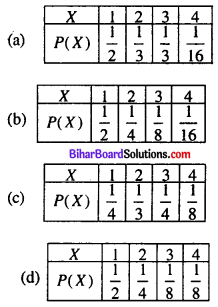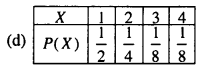Question 26.
Suppose that two cards are drawn at random from a deck of cards. Let X be the number of aces obtained. Then, the value of E(X) is
(a) $$\frac{37}{221}$$
(b) $$\frac{5}{13}$$
(c) $$\frac{1}{13}$$
(d) $$\frac{2}{13}$$
(d) $$\frac{2}{13}$$

Question 27.
The random variable X can take only the values 0, 1, 2. Given that, P(X = 0) = P (X = 1) = p and that E(X2) = E(X), find the value of p.
(a) $$\frac{1}{5}$$
(b) $$\frac{3}{10}$$
(c) $$\frac{2}{5}$$
(d) $$\frac{1}{2}$$
(d) $$\frac{1}{2}$$

Question 28.
The variance and standard deviation of the number of heads in three tosses of a coin are respectively
(a) $$\frac{3}{4}, \frac{\sqrt{3}}{2}$$
(b) $$\frac{1}{4}, \frac{1}{2}$$
(c) $$\frac{3}{4}, \frac{\sqrt{3}}{4}$$
(d) None of these
(d) None of theseQuestion 29.
In a meeting, 70% of the members favour and 30% oppose a certain proposal, A member is selected at random and we take X = 0, if opposed and X = 1, if he is in favour. Then, E(X) and Var(X) are respectively
(a) $$\frac{3}{7}, \frac{5}{17}$$
(b) $$\frac{13}{15}, \frac{2}{15}$$
(c) $$\frac{7}{10}, \frac{21}{100}$$
(d) $$\frac{7}{10}, \frac{23}{100}$$
(c) $$\frac{7}{10}, \frac{21}{100}$$

Question 30.
For the following probability distribution, the standard deviation of the random variable X is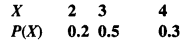(a) 0.5
(b) 0.6
(c) 0.61
(d) 0.7
(d) 0.7

Question 31.
The variance of random variable X i.e. $$\sigma_{x}^{2}$$ or var (X) is equal to
(a) E(X2) + [E(X2)2]2
(b) E(X) – [E(X2)]
(c) E(X2) – [E(X)]2
(d) None of these
(c) E(X2) – [E(X)]2

Question 32.
A coin is biased so that the head is 3 times likely to occur as a tail. If the coin is tossed twice, then find the probability distribution of the number of tails.Question 33.
A pair of the die is thrown 4 times. If getting a doubled is considered a success, then find the probability distribution of a number of successes.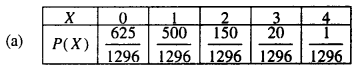Question 34.
Find the probability of throwing atmost 2 sixes in 6 throws of a single die.
(a) $$\frac{35}{18}\left(\frac{5}{6}\right)^{3}$$
(b) $$\frac{35}{18}\left(\frac{5}{6}\right)^{4}$$
(c) $$\frac{18}{29}\left(\frac{2}{3}\right)^{4}$$
(d) $$\frac{18}{29}\left(\frac{2}{3}\right)^{3}$$
(b) $$\frac{35}{18}\left(\frac{5}{6}\right)^{4}$$

Question 35.
A die is thrown again and again until three sixes are obtained. Find the probability of obtaining third six in the sixth throw of the die.
(a) $$\frac{625}{23329}$$
(b) $$\frac{621}{25329}$$
(c) $$\frac{625}{23328}$$
(d) $$\frac{620}{23328}$$
(c) $$\frac{625}{23328}$$Question 36.
Ten eggs are drawn successively with replacement from a lot containing 10% defective eggs. Then, the probability that there is atleast one defective egg is
(a) $$1-\frac{7^{10}}{10^{10}}$$
(b) $$1+\frac{7^{10}}{10^{10}}$$
(c) $$1+\frac{9^{10}}{10^{10}}$$
(d) $$1-\frac{9^{10}}{10^{10}}$$
(d) $$1-\frac{9^{10}}{10^{10}}$$

Question 37.
The probability of a man hitting a target is $$\frac{1}{4}$$. How many times must he fire so that the probability of his hitting the target at least once is greater than $$\frac{2}{3}$$?
(a) 4
(b) 3
(c) 2
(d) 1
(a) 4

Question 38.
Eight coins are thrown simultaneously. Find the probability of getting atleast 6 heads.
(a) $$\frac{31}{128}$$
(b) $$\frac{37}{256}$$
(c) $$\frac{37}{128}$$
(d) $$\frac{31}{256}$$
(b) $$\frac{37}{256}$$

Question 39.
A bag contains 6 red, 4 blue and 2 yellow balls. Three balls are drawn one by one with replacement. Find the probability of getting exactly one red ball.
(a) $$\frac{1}{4}$$
(b) $$\frac{3}{8}$$
(c) $$\frac{3}{4}$$
(d) $$\frac{1}{2}$$
(b) $$\frac{3}{8}$$

Question 40.
Eight coins are thrown simultaneously. What is the probability of getting atleast 3 heads?
(a) $$\frac{37}{246}$$
(b) $$\frac{21}{256}$$
(c) $$\frac{219}{256}$$
(d) $$\frac{19}{246}$$
(c) $$\frac{219}{256}$$Question 41.
If the chance that a ship arrives safely at a port is $$\frac{9}{10}$$; find the chance that out of 5 expected ships, atleast 4 will arrive safely at the port.
(a) $$\frac{91854}{100000}$$
(b) $$\frac{32805}{100000}$$
(c) $$\frac{59049}{100000}$$
(d) $$\frac{26244}{100000}$$
(a) $$\frac{91854}{100000}$$

Expanding binomials calculator. This calculators lets you calculate expansion of a binomial in your website.

Question 42.
If the mean and the variance of a binomial distribution are 4 and, then find P(X ≥ 1).
(a) $$\frac{720}{729}$$
(b) $$\frac{721}{729}$$
(c) $$\frac{728}{729}$$
(d) $$\frac{724}{729}$$
(c) $$\frac{728}{729}$$

Question 43.
A pair of dice is thrown 200 times. If getting a sum of 9 is considered a success, then find the mean and the variance respectively of the number of successes.
(a) $$\frac{400}{9}, \frac{1600}{81}$$
(b) $$\frac{1600}{81}, \frac{400}{9}$$
(c) $$\frac{1600}{81}, \frac{200}{9}$$
(d) $$\frac{200}{9}, \frac{1600}{81}$$
(b) $$\frac{1600}{81}, \frac{400}{9}$$

Question 44.
In a binomial distribution, the sum of its mean and variance is 1.8. Find the probability of two successes, if the event was conducted times.
(a) 0.2623
(b) 0.2048
(c) 0.302
(d) 0.305
(b) 0.2048Question 45.
If the sum and the product of the mean and variance of a binomial distribution are 24 and 128 respectively, then find the distribution.
(a) $$\left(\frac{1}{4}+\frac{3}{4}\right)^{32}$$
(b) $$\left(\frac{1}{2}+\frac{1}{2}\right)^{30}$$
(c) $$\left(\frac{1}{2}+\frac{1}{2}\right)^{32}$$
(d) $$\left(\frac{1}{4}+\frac{3}{4}\right)^{30}$$
(c) $$\left(\frac{1}{2}+\frac{1}{2}\right)^{32}$$

Question 46.
If the sum of the mean and variance of a binomial distribution is 15 and the sum of their squares is 17, then find the distribution.
(a) $$\left(\frac{2}{3}+\frac{1}{3}\right)^{25}$$
(b) $$\left(\frac{1}{2}+\frac{1}{2}\right)^{25}$$
(c) $$\left(\frac{1}{2}+\frac{1}{2}\right)^{27}$$
(d) $$\left(\frac{2}{3}+\frac{1}{3}\right)^{27}$$
(d) $$\left(\frac{2}{3}+\frac{1}{3}\right)^{27}$$

Question 47.
The mean and the variance of a binomial distribution are 4 and 2 respectively. Find the probability of atleast 6 successes.
(a) $$\frac{37}{256}$$
(b) $$\frac{32}{255}$$
(c) $$\frac{34}{259}$$
(d) $$\frac{31}{256}$$
(a) $$\frac{37}{256}$$

Question 48.
If P(A ∩ B) = $$\frac{7}{10}$$ and P(b) = $$\frac{17}{20}$$, P(A|B) equals
(a) $$\frac{14}{17}$$
(b) $$\frac{17}{20}$$
(c) $$\frac{7}{8}$$
(d) $$\frac{1}{8}$$
(a) $$\frac{14}{17}$$

Question 49.
If P(A) = $$\frac{3}{10}$$, P(b) = $$\frac{2}{5}$$ and P(A ∪ B) = $$\frac{3}{5}$$, then P(B|A) + P(A|B) equals
(a) $$\frac{1}{4}$$
(b) $$\frac{1}{3}$$
(c) $$\frac{5}{12}$$
(d) $$\frac{7}{12}$$
(d) $$\frac{7}{12}$$Question 50.
If P(A) = $$\frac{2}{5}$$, P(B) = $$\frac{3}{10}$$ and P(A ∩ B) = $$\frac{1}{5}$$, then P(A’|B’) . (P(B’|A’) is equal to
(a) $$\frac{5}{6}$$
(b) $$\frac{5}{7}$$
(c) $$\frac{25}{42}$$
(d) 1
(b) $$\frac{5}{7}$$

Question 51.
If A and B are two events sue that P(A) = $$\frac{1}{2}$$, P(b) = $$\frac{1}{3}$$, P(A|B) = $$\frac{1}{4}$$ then (A’ ∩ B’) equals
(a) $$\frac{1}{12}$$
(b) $$\frac{3}{4}$$
(c) $$\frac{1}{4}$$
(d) $$\frac{3}{16}$$
(c) $$\frac{1}{4}$$

Question 52.
If P(A) = 0.4, P(b) = 0.8 and P(B|A) = 0.6, then P(A ∪ B) equal to
(a) 0.24
(b) 0.3
(c) 0.48
(d) 0.96
(c) 0.48

Question 53.
If A and B are two events and A ≠ Φ, B ≠ Φ, then
(a) P(A|B) = P(A) . P(b)
(b) P(A|B) = $$\frac{P(A \cap B)}{P(B)}$$
(c) P(A|B) . P(B|A) = 1
(d) P(A|B) = P(A)|P(b)
(b) P(A|B) = $$\frac{P(A \cap B)}{P(B)}$$

Question 54.
A and B are events such that P(A) = 0.4, P(b) = 0.3 and P(A ∪ B) = 0.5. Then P(B’ ∩ A) equals
(a) $$\frac{2}{3}$$
(b) $$\frac{1}{2}$$
(c) $$\frac{3}{10}$$
(d) $$\frac{1}{5}$$
(d) $$\frac{1}{5}$$Question 55.
You are given that A and B are two events such that P(b) = $$\frac{3}{5}$$, P(A|B) = $$=\frac{4}{5}$$, then P(A) equals
(a) $$\frac{3}{10}$$
(b) $$\frac{1}{5}$$
(c) $$\frac{1}{2}$$
(d) $$\frac{3}{5}$$
(c) $$\frac{1}{2}$$

Question 56.
If P(b) = $$\frac{3}{5}$$, P(A|B) = $$\frac{1}{2}$$ and P(A ∪ B) = $$\frac{4}{5}$$, then P(A ∪ B’) + P(A’ ∪ B) = 1
(a) $$\frac{1}{5}$$
(b) $$\frac{4}{5}$$
(c) $$\frac{1}{2}$$
(d) 1
(d) 1

Question 57.
If A and Bare two independent events with P(A) = $$\frac{3}{5}$$ and P(b) = $$\frac{4}{9}$$, then P(A’ ∩ B’) equals
(a) $$\frac{4}{15}$$
(b) $$\frac{8}{45}$$
(c) $$\frac{1}{3}$$
(d) $$\frac{2}{9}$$
(d) $$\frac{2}{9}$$

Question 58.
If the events A and B are independet, then P(A ∩ B) is equal to
(a) P(A) + P(b)
(b) P(A) – P(b)
(c) P(A) . P(b)
(d) P(A) | P(b)
(c) P(A) . P(b)Question 59.
Two events E and F are independent. If P(E) = 0.3, P(E ∪ F) = 0.5, then P(E|F) – P(F|E) equals
(a) $$\frac{2}{7}$$
(b) $$\frac{3}{35}$$
(c) $$\frac{1}{70}$$
(d) $$\frac{1}{7}$$
(c) $$\frac{1}{70}$$

Question 60.
A bag contains 5 red and 3 blue balls. If 3 balls are drawn at random without replecement the probability of getting exactly one red ball is
(a) $$\frac{45}{196}$$
(b) $$\frac{135}{392}$$
(c) $$\frac{15}{56}$$
(d) $$\frac{15}{29}$$
(c) $$\frac{15}{56}$$

Question 61.
A die is thrown and card is selected a random from a deck of 52 playing cards. The probability of gettingan even number on the die and a spade card is
(a) $$\frac{1}{2}$$
(b) $$\frac{1}{4}$$
(c) $$\frac{1}{8}$$
(d) $$\frac{3}{4}$$
(c) $$\frac{1}{8}$$

Question 62.
A box contains 3 orange balls, 3 green balls and 2 blue balls. Three balls are drawn at random from the box without replacement. The probability of drawing 2 green balls and one blue ball is
(a) $$\frac{3}{28}$$
(b) $$\frac{2}{21}$$
(c) $$\frac{1}{28}$$
(d) $$\frac{167}{168}$$
(a) $$\frac{3}{28}$$

Question 63.
A flashlight has 8 batteries out of which 3 are dead. If two batteries are selected without replacement and tested, the probability that both are deal is
(a) $$\frac{33}{56}$$
(b) $$\frac{9}{64}$$
(c) $$\frac{1}{14}$$
(d) $$\frac{3}{28}$$
(d) $$\frac{3}{28}$$Question 64.
Two dice are thrown. If it is known that the sum of numbers on the dice was less than 6, the probability of getting a sum 3, is
(a) $$\frac{1}{18}$$
(b) $$\frac{5}{18}$$
(c) $$\frac{1}{5}$$
(d) $$\frac{2}{5}$$
(c) $$\frac{1}{5}$$

Question 65.
Two cards are drawn from a well shuffled deck of 52 playing cards with replacement. The probability, that both cards are queens, is
(a) $$\frac{1}{13} \times \frac{1}{13}$$
(b) $$\frac{1}{13}+\frac{1}{13}$$
(c) $$\frac{1}{13} \times \frac{1}{17}$$
(d) $$\frac{1}{13} \times \frac{4}{51}$$
(a) $$\frac{1}{13} \times \frac{1}{13}$$

Question 66.
The probability of guessing correctly at least 8 out of 10 answers on a true-false type examiniation is
(a) $$\frac{7}{64}$$
(b) $$\frac{7}{128}$$
(c) $$\frac{45}{1024}$$
(d) $$\frac{7}{41}$$
(b) $$\frac{7}{128}$$

Question 67.
The probability distribution of a discrete random variable X is given below: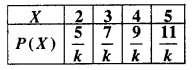The value of k is
(a) 8
(b) 16
(c) 32
(d) 48
(c) 32

Question 68.
For the following probability distribution: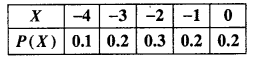E(X) is equal to
(a) 0
(b) -1
(c) -2
(d) -1.8
(d) -1.8

Question 69.
For the following probability distributionE(X2) is equal to
(a) 3
(b) 5
(c) 7
(d) 10
(d) 10

Question 70.
Suppose a random variable X follows the binomial distribution with parameters n and p, where 0 < p < 1. If p(x = r) / P(x = n – r) is dindependent of n and r, then p equals
(a) $$\frac{1}{2}$$
(b) $$\frac{1}{3}$$
(c) $$\frac{1}{5}$$
(d) $$\frac{1}{7}$$
(a) $$\frac{1}{2}$$Question 71.
A box has 100 pens of which 10 are defective. What is the probability that out of a sample of 5 pens drawn one by one with replacement at most one is defective?
(a) $$\left(\frac{9}{10}\right)^{5}$$
(b) $$\frac{1}{2}\left(\frac{9}{10}\right)^{4}$$
(c) $$\frac{1}{2}\left(\frac{9}{10}\right)^{5}$$
(d) $$\left(\frac{9}{10}\right)^{5}+\frac{1}{2}\left(\frac{9}{10}\right)^{4}$$
(d) $$\left(\frac{9}{10}\right)^{5}+\frac{1}{2}\left(\frac{9}{10}\right)^{4}$$